Hunt, J. F., Zhang, H., and Huang, Y. (2015). "Analysis of cantilever-beam bending stress relaxation properties of thin wood composites," BioRes. 10(2), 3131-3145.

#### Abstract

An equivalent strain method was used to analyze and determine material relaxation properties for specimens from particleboard, high density fiberboard, and medium density fiberboard. Cantilever beams were clamped and then deflected to 11 m and held for either 2 h or 3 h, while the load to maintain that deflection was measured vs. time. Plots of load relaxation for each specimen showed similar load relaxation vs. time even though there were some slight differences in the maximum load per sample. Three models were developed to fit the relaxation data. The first model was a simple log decrement. This simple log model used only one variable, the relaxation coefficient, to describe the relaxation behavior as the log of time. The log decrement model was marginal at best in modeling the relaxation data. The second and third models, however, used equivalent strain methods. The second model assumed a combined linear-elastic spring and a Kelvin-Voigt spring-dashpot model. The third model used a combination of a linear-elastic spring (linear strain) element, a Kelvin-Voigt (spring-dashpot, visco-elastic strain) element, and a dashpot (permanent strain) element for its total configuration. Both equivalent strain models provided excellent correlations for the two lengths of time used for this series. Estimated mechanical and relaxation, or creep properties, were determined from the equivalent strain method using cantilever beam equations.

#### Full Article

Analysis of Cantilever-Beam Bending Stress Relaxation Properties of Thin Wood Composites

John F. Hunt,a,* Houjiang Zhang,and Yan Huang b

An equivalent strain method was used to analyze and determine material relaxation properties for specimens from particleboard, high density fiberboard, and medium density fiberboard. Cantilever beams were clamped and then deflected to 11 m and held for either 2 h or 3 h, while the load to maintain that deflection was measured vs. time. Plots of load relaxation for each specimen showed similar load relaxation vs. time even though there were some slight differences in the maximum load per sample. Three models were developed to fit the relaxation data. The first model was a simple log decrement. This simple log model used only one variable, the relaxation coefficient, to describe the relaxation behavior as the log of time. The log decrement model was marginal at best in modeling the relaxation data. The second and third models, however, used equivalent strain methods. The second model assumed a combined linear-elastic spring and a Kelvin-Voigt spring-dashpot model. The third model used a combination of a linear-elastic spring (linear strain) element, a Kelvin-Voigt (spring-dashpot, visco-elastic strain) element, and a dashpot (permanent strain) element for its total configuration. Both equivalent strain models provided excellent correlations for the two lengths of time used for this series. Estimated mechanical and relaxation, or creep properties, were determined from the equivalent strain method using cantilever beam equations.

Keywords: Thin wood composites; Cantilever-beam bending; Stress relaxation; Relaxation coefficient; Equivalent strain; Kelvin model; Burgers model; Elastic strain; Visco-elastic strain; Permanent strain

Contact information: a: USDA Forest Products Laboratory, 1 Gifford Pinchot Drive, WI 53726-2398, USA; b: School of Technology, Beijing Forestry University, Beijing 100083, China;

* Corresponding author: jfhunt@fs.fed.us

INTRODUCTION

Thin wood composites, as described in this paper, include such products as fiberboard, particleboard, laminated veneer, and pulp molded products that measure between 1 to 5 mm (Zhang et al. 2010). These composite materials can be used widely for products such as box boards, container boards, non-load-bearing building panels of building materials, architectural panels, and decorated panels. The performance or structural needs are different for each application that requires an understanding of the mechanical properties of these wood composites for optimal utilization. The mechanical properties of thin wood composites include short term performance properties, such as static elastic modulus, dynamic elastic modulus, storage modulus, loss modulus, and shear modulus. Determining these mechanical properties for thin wood composites is important for characterization during panel production, as well as for analysis for potential applications.

Equally important is the analysis of long-term performance properties, such as stress relaxation and creep response. Stress relaxation of wood and wood materials has been investigated (Schniewind and Richmond 1968; Cheng 1985; Larson 1999; Feng and Zhao 2010). Three types of long-term test methods have been used that have included the tension test (Xie and Zhao 2004; Cao et al. 2006), the mid-point bending test (Ikuho et al. 2002), and the dual cantilever bending test (Ebrahimzadeh and Kubat 1993). While most tests use the constant load method to measure strain as a function of time (creep), there are a few that use constant displacement and measure the resultant load as a function of time (stress relaxation).

Analysis of creep and stress relaxation for wood and wood composite ranges from very simple models that are empirically fit models, to full mechanistic models. Simple models that usually have only one variable are generally inadequate to describe the non-linear behavior (Smulski 1989). The simple models either under- or over-characterize the initial response, followed by over- or under-characterizing the long-term creep or stress relaxation behavior, respectively. More complex (non-linear) models, even those that are used by other non-wood industries, have been tried with good success to model non-linear data for wood, OSB, and laminated timber (Smulski 1989; Cai et al. 2002). Some models use power law and exponential fitting equations, but they have limited correlation with the wood material. Cai et al. (2002) showed that improved fits were obtained with additional empirically fit variables providing a new power model. However, they found that while added variables provide excellent fit to the data, they may not necessarily relate to physical properties or characteristics.

Mechanistic viscoelastic models have been shown to provide both excellent fit to the data, while also providing variables that correspond to physical properties of the material, even non-wood edible materials (Chandra and Sobral 2000). The use of various combinations of elements of springs and dashpots that relate to stress/strain relationships, provides the foundation for different modeling options and their equations. When applied to wood, these mechanistic equations provide both excellent curve fitting of the data, and potential understanding of the material properties and their response to loads (Moutee et al. 2007). The cited authors showed that a rheological (mechanistic) model could be used to accurately predict response of a constantly loaded cantilever wooden beam during drying. The basis of the model was a combination of mechanistic elements that experienced the same stress, but responded differently (strain response), corresponding to the different elements of the model.

This paper aims to use the mechanistic modeling method for determining stress relaxation properties of thin wood composites based on the cantilever beam bending theory. The test is not a constant load test representing creep response, but uses constant displacement representing stress relaxation of the cantilever beam, and the associated properties to describe the response.

Stress Relaxation Equations for a Cantilever Beam

As with all visco-elastic materials, stress relaxation or extension occurs as soon as a load is applied. For straight in-line constant tensile or compression loading, stress remains constant while strain movement occurs, which is related to the viscous response to that load. If a visco-elastic material were loaded in-line loading to a specific displacement and then held in that position, then the total movement or total strain would remain the same, while stress would decrease in response to the material’s viscous characteristic, thus decreasing total stress. The curves of constant strain and decreasing stress rate are shown in Fig. 1 (Feng and Zhao 2010). Similarly, when a visco-elastic beam is loaded with a constant load, it will continue to deflect as the material relaxes due to visco-strain behavior. For simple beam bending analyses, it is assumed that the extension on the top and bottom of the beams have similar visco-behavior in both tension (bottom) as with compression (top) within initial loading conditions. Therefore existing beam bending equations can be used to describe the stresses and strains within the beam. The simply supported beam, as well as the cantilever beam, have non-uniform stress distributions through the thickness as well as along the length of the beam. For simple analyses for wood composite materials in general one assumes that the material properties are similar throughout the thickness. However, it is known that most wood composites have a non-uniform density profile that affects the static material properties. Thus it is reasonable to assume that there will be similar non-uniform visco-elastic properties with respect to the thickness. The non-uniform material properties relative to the thickness dimension will not be addressed in this paper.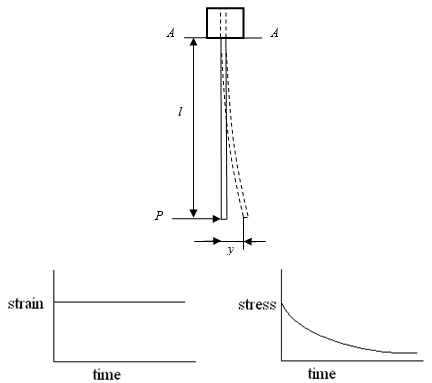Fig. 1. Cantilever beam constant strain due to constant end-beam displacement and resulting stress relaxation of wood-based composite materials

For a cantilever beam, beam deflection at the end of the beam, Fig. 1, can be determined from Eq. 1. If the cantilever beam is deflected to a known displacement, y, and the load, Pmax, is measured, then by rearranging Eq. 1, the static bending modulus of elasticity, Ee, can be determined,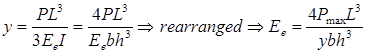(1)

where y is the end deflection (m), Ee is the bending modulus of elasticity (N/m2), is the end-point load (N), is the cantilever beam length (m), is the area moment of inertia (m4), b is the base width of the specimen (m), and h is the thickness of the specimen (m).

At the known deflection, y, the maximum bending stress can be calculated using Eq. 2 (Liu 2004). The maximum stress is at the cantilever beam clamp, where x=0, and minimum stress at the cantilever end, where x=L. Stress decreases linearly, starting at the clamp and decreases to zero at the deflected end. The average stress for the entire beam is then half the maximum stress.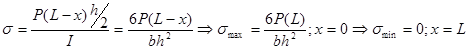(2)

There are several models that have been used to describe the response over time for visco-elastic materials. The Burgers viscoelastic model, Fig. 2, is a combination of three elements that include: a linear elastic spring, a dash-pot, and a combined spring plus dash-pot or a Kelvin-Voigt element (Chandra and Sobral 2000). Based on the stress response,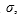from the initial cantilever beam tip displacement, the total strain,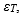equals the strain response as measured by the load decrease as a result of the beam relaxation.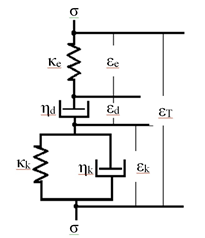Fig. 2. Burgers viscoelastic stress-strain model is a combination of a linear elastic spring element, a dashpot element, and combined spring plus dashpot element (Kelvin-Voight model)

The total strain,is equal to the sum of the elastic strain (spring),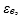plus the permanent strain (dashpot),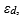and the viscoelastic strain (Kelvin-Voigt model),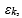as shown in Fig. 2, and described in Eq. 3. For this paper, we assume homogenous material properties; however, additional research would be needed to more accurately determine differences in stress-strain behavior based on variations along the beam, variations of density through the thickness of the beam, and variations in fiber or particle alignment.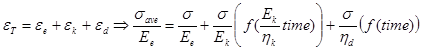(3)

The elastic modulus, Ee, was determined from the maximum load for a given displacement at time equal to zero using Eq. 1. According to Eq. 2, the stress along the length of the beam decreases linearly from maximum at the clamp to zero at the beam’s end and that the strain response was also linear with stress, and thus we used the average stress,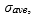Eq. 4 for our total strain calculations.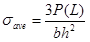(4)

The elastic element of the model maintains a linear relationship with the varying load, and responds such that elastic strain decreases as the load decreases. The Kelvin-Voigt element of the model produces a delayed visco-elastic response under load, where the tensile side of the beam continues to extend because of the tension stress, and the compression side continues to compress due to the compression stress, thus yielding a lowering in the overall cantilever beam load as a function of time. As the load decreases, the strain due to the elastic portion decreases, but the strain due to relaxation increases, so the total strain remains the same. The permanent displacement strain was also taken into consideration based on an estimated permanent relaxation response over time. The sum of the strains from each section equals the total initial strain.

The total strain, εT, equation is given by Eq. 5 and each of the elements of the model are given in Eq. 6,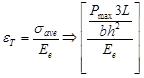(5)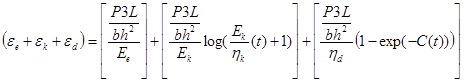(6)

where Ek is the viscous modulus for the visco-elastic Kelvin model (N-m-2), ηk is the relaxation coefficient for the visco-elastic Kelvin model (N-m-2-time), ηd is the relaxation coefficient for the permanent displacement dashpot (N-m-2-time), and C is the time constant coefficient.

The components of stress, fraction Eq. 7, were removed from Eqs. 5 and 6 and simplified in Eqs. 8a and 8b to obtain the inverse of load, 1/P, as shown in Eq. 8c.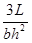(7)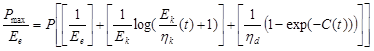(8a)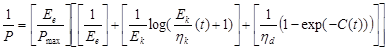(8b)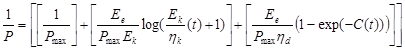(8c)

To solve the load relaxation behavior as a function of time, six variables need to be determined; PmaxEeEkηkηd, and CPmax can be determined from the initial load to a known displacement, yEe can be determined from Eq. 1 and the total strain, and εT, can be determined from Eq. 5. Assuming the Burgers model is correct, the remaining four variables can be obtained from a curve fitting program from the known load vs. time curve. The load vs. time curve is the combination of all three (or more) representative components to the relaxation response. Additional data, such as a measurement of immediate spring-back displacement (elastic) after unloading the beam, the spring-back-relaxation displacement as a function of time (visco-elastic), and the final displacement (permanent) can be used to help determine these unknown better relaxation characteristics. Also, various load levels should be used to test whether the relaxation characteristics exhibit linear or non-linear behavior as a function of load or stress level. These tests are beyond the scope of this initial research paper but should be done to further understand the complex composite relaxation behavior using the cantilever beam.

A simplified elastic element plus the Kelvin-Voigt element (Burgers model without a dashpot) might also be useful to analyze stress relaxation response where loading conditions are minimal and where permanent displacement may not have as significant of an influence on total strain. Elastic modulus, Ee, was still calculated from Eq. 1, and total strain was calculated from Eq. 5. The permanent strain effect in Eq. 8c was removed, as shown in Eq. 9, to model an elastic plus a Kelvin-Voigt model. Now, only two variables need to be determined using this equation, Ek and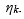It is easier to determine potential characteristics through curve fitting the load data when only two variables are needed. Using this simpler model may provide initial information or a first approach to determine initial ball-park visco-elastic constants. Extensive investigation with this model was also beyond the scope of this paper, but should be done in further research.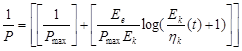(9)

A single variable model has also been used by some to describe the overall relaxation response that uses one variable, called a relaxation coefficient (Cheng 1985). Cheng used the equation and found good agreement with wood specimens. The equation is based on the log decrement as a function of time fit to wood creep data, Eq. 10. The result is one relaxation coefficient, A, that can be used to describe the load relaxation behavior, but it is not tied to any mechanical properties of the panel.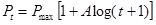(10)

EXPERIMENTAL

Test Apparatus

A new test vertical cantilever beam apparatus was built based on the cantilever beam bending load and deformation theory (Zhang et al. 2010). The test apparatus includes two main parts: the mechanical structure part and the data processing part. The components of the apparatus are shown in Fig. 3 (a), and the apparatus photo is shown in Fig. 3 (b).

The specimen is clamped in a hanging position. A static displacement was made by displacing the end of the specimen to a specific distance using an end hook. A load cell is attached to the hook that measures the load applied at the end of the beam. A laser displacement gage is used to determine initial and displacement of the beam end.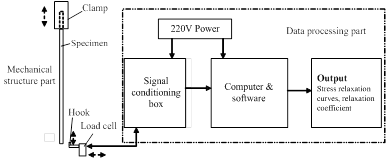(a) Components diagram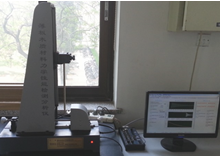(b) Photo

Fig. 3. Cantilever beam test apparatus

Materials and Testing

This was the first stress relaxation study on this apparatus. The following three kinds of specimens were used: 3.7-mm-thick high-density fiberboard (HDF), 4.6-mm-thick medium-density fiberboard (MDF), and 5.0-mm-thick particleboard (PB), as can be seen in Table 1. The specimens were clamped at 50 mm on one end. The total beam lengths of the specimens were l+50 mm. Maximum bending strength was not obtained for this study. Specimens were tested in normal laboratory conditions around 20 °C. Exact relative humidity (RH) conditions were not available at the time of tests. All samples were tested under similar RH conditions.

Table 1. Type, Size, Symbol, and Number of the Specimens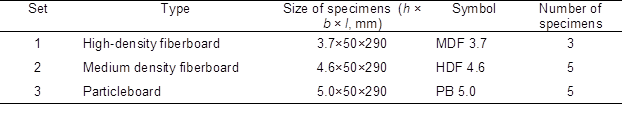Figure 3 shows the specimen clamped as a vertical cantilever beam. A perpendicular hook at the free end was used to set the initial distance at the end of the specimen, y, resulting in a displacement load, P, recorded at the base of the hook. The maximum load was recorded at a set displacement of 20 mm. The hook remained on the end of the specimen, and load was measured as a function of time. The initial bending stress was set to approximately 10% to 20% of the modulus of rupture (MOR) for three materials.

RESULTS AND DISCUSSION

Test Results

The load vs. time data were analyzed using Tablecurve 2D software (Systat Software, Inc, San Jose, CA, USA) to curve fit the data based on the three models. Beam load was recorded as a function of time, after an initial 11 mm beam displacement applied at the free end and held in position for 2 or 3 h. With the beam held at a constant position, the load data showed a gradual decrease (relaxation) with time. While the displacement was the same for all boards, there were slight differences in time for the maximum load to reach the 11 mm displacement. For each specimen, the initial load, Pmax, at time = 0 was used to calculate a static modulus of elasticity, MOE or Ee, using Eq. 1, and listed in Table 2.

For visual comparison purposes, the load relaxation for each board were normalized to 100% of the maximum and are shown in Fig. 4. Four specimens were held for 2 h, and the 5thspecimen was held for 3 h. The load vs. time data were then input into the analysis software to determine the variables for the three models. The results are listed in Tables 2 and 3. After the tests, the specimens retained a slight curved shape (permanent displacement) as a result of creep and stress relaxation within the boards.

The authors are aware that environmental test conditions such as temperature and relative humidity (equilibrium moisture content, EMC) have significant effects on performance characteristics. These need to be addressed to determine real world long term loading effects. For this initial study, however, temperature and EMC were beyond the scope of the investigation and were not considered as part of the equation. These effects should be considered as part of future mechanistic equations and study.

Coefficients of determination, r2, for each of the fitted models are listed in Tables 2 and 3. When using the simple model, the relaxation model correlated marginally with the data for the length of time tested for these series of specimens, for either 7,200 or 10,800 s. The simple model over-estimated the initial relaxation, followed by under-estimating the load relaxation as time increased, as shown in Fig. 5. The load relaxation coefficient, A, varied from board to board, from a low of 0.0279 to a high of 0.0518, and it did not seem to have any specific trends except for being slightly lower for the MDF specimens than the other two board series. It is hard to place any significance on these differences because there are several other variables including thickness and material properties that compound the response to stress relaxation.

Table 2 also shows the results when using the combined elastic plus Kelvin-Voigt elements. Table 3 shows the results from the Burgers model. The coefficients of determination for both models were very good, ranging from 0.984 to 0.999, and between 0.995 and 0.999, respectively.

Both models showed reasonably similar visco-elastic modulus, Ek, values. Plotting Ek (not shown) shows a relative linear relationship where the Burger’s visco-elastic modulus was approximately 1.35 times the elastic plus Kelvin-Voight’s visco-elastic modulus. In this initial determination, it is important not to confuse visco-elasticity, Ek, with conventional modulus of elasticity,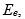values. The Ek values calculated in this paper represent interaction with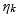values but primarily correlates to an elastic portion of the visco-elastic element.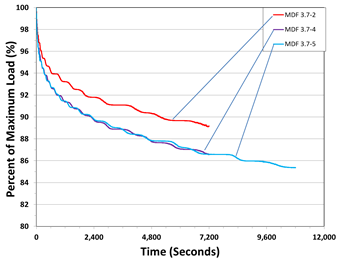(a)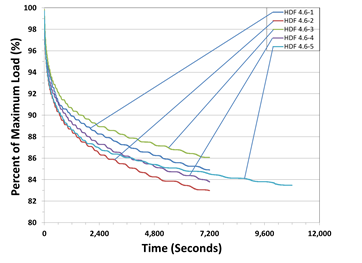(b)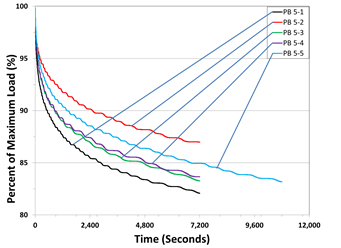(c)

Fig. 4. Percent of maximum load vs. time for (a) medium-density fiberboard (MDF) 3.7 mm thick series; (b) high-density fiberboard (HDF) 4.6 mm thick series; (c) particleboard (PB) 5.0 mm thick series held at a constant displacement

The coefficients of viscosity, ηk, for the Burgers model was approximately 0.27 times that of the elastic plus Kelvin-Voigt model. The lower values could be due to the addition of the permanent displacement dash-pot element in the Burgers model. The visco-elastic dashpot of the Kelvin-Voight element was required to account for all the difference associated with relaxation both viscous and permanent. From Fig. 5 (a) and (c), it can be seen that the elastic plus Kelvin-Voight model started to diverge from the actual load relaxation, while the Burger’s model followed relaxation very well. Table 4 shows for each panel, the estimated strain percent for each element in the Burgers model after load for either 7,200 or 10,800 seconds. The permanent displacement strain was estimated to be between 0.21 and 4.1 percent of the total at these load conditions. Figure 6 shows the curves for PB 5-5, but these plots represent similar results for the percent total strains for the other sample beams as a function of time. Both visco-elastic strain percent and the permanent strain percent represents the relaxation response over time and are shown to increase, while the elastic strain decreases as load also decreases. Adding all the strains together equals the total initial strain from the initial beam displacement.

Table 2. Initial Load and Elastic Properties for 11-mm End Beam Displacement*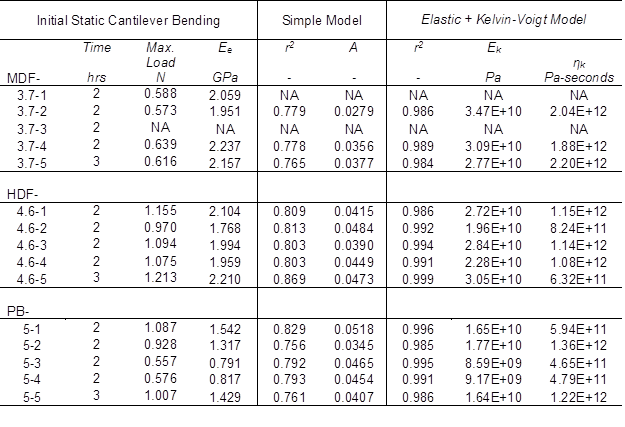Ee = Cantilever beam Static Elastic Modulus

A = Relaxation coefficient

Ek = Visco-Modulus; not to be confused with Ee, Elastic Modulus

ηk = Relaxation coefficient for the visco-elastic Kelvin

*Correlations and test variables determined from the simple and elastic plus Kelvin-Voigt models to fit the load data for both 7,200 and 10,800 s relaxation tests

Table 3. Curve Fit Correlations and Test Variables Determined for the Burgers Model to Fit the Load Relaxation Data for Both 2- and 3-Hour Relaxation Tests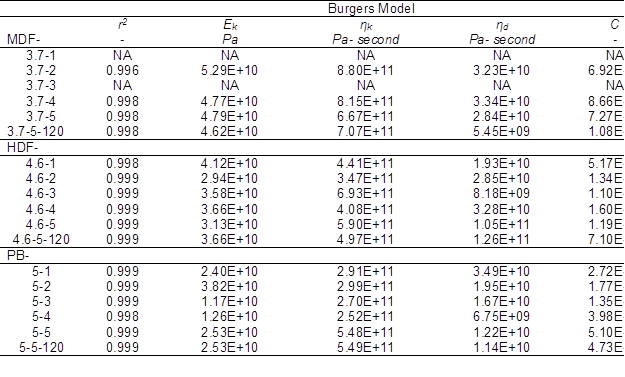Ek = Visco-Modulus; not to be confused with Ee, Elastic Modulus

ηk = Relaxation coefficient for the visco-elastic Kelvin

ηd = Relaxation coefficient for the permanent displacement dashpot

C = Time constant coefficient.

Table 4. Strain Percent for Each of the Burgers Model; Elastic, Visco-Elastic, and Permanent Elements of the Estimated Stress Relaxation Strains after either 7,200 seconds (specimens 1 – 4) or 10,800 seconds (specimen 5)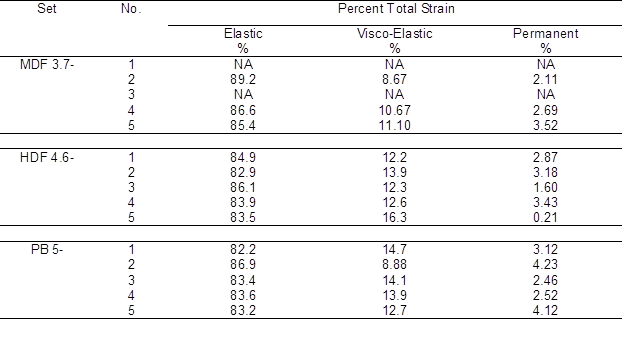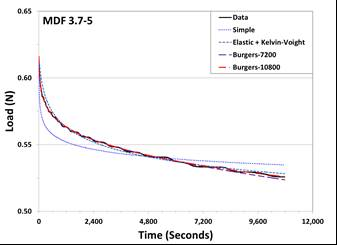(a)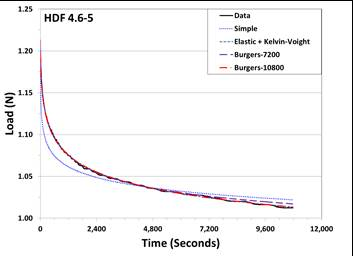(b)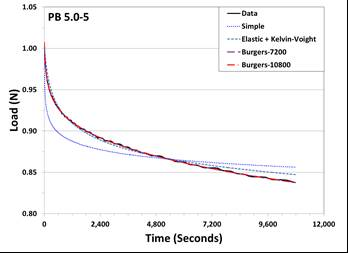(c)

Fig. 5. Load relaxation load data plotted with the simple, Elastic plus Kelvin-Voigt, and Burgers models for the specimens tested for 3 hours and the estimates calculated at 7,200 and 10,800 seconds for: (a) medium density fiberboard (MDF) 3.7 mm thick series; (b) high density fiberboard (HDF) 4.6 mm thick series; and (c) particleboard (PB) 5.0 mm thick series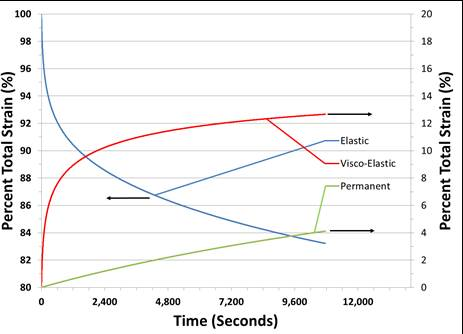Fig. 6. Percent total strain for each of the three elements of the Burgers strain model for specimen PB 5-5: a) elastic (spring) element; b) Kelvin-Voigt (combined spring and dashpot) element; and c) permanent (dashpot) element. Total of all three equals 100%

While the total relaxation times used for this initial creep study were limited to 2 h (7200 seconds) and 3 h (10800 seconds), the times were sufficient to show the potential of the model’s ability to model the relaxation behavior, but lacked the length of time or the necessary additional information to accurately determine all the variables listed in the elastic plus Kelvin-Voigt model or the Burgers model. One or more additional tests using the same set-up would be necessary to help solve for all of the variables. One such test would be, after a set time, to release the beam from constant displacement, and allow the beam to spring back. The new displacement would be recorded as a function of time during its response to the initial elastic spring-back followed by creep recovery over time, ending in permanent displacement. These values and recovery rates could then be used to better solve for the permanent relaxation displacement dashpot and the Kelvin-Voigt dashpot rates. Thus, the cantilever beam could be used to also measure relaxation rates of composite panels. This study shows the potential of using a mechanistic approach to modeling the behavior of the cantilever beam using constant displacement relaxation.

CONCLUSIONS

A simple visco-elastic model was not able to adequately model the load (loss) vs. time stress relaxation behavior of the three panel types used for this study. The elastic plus Kelvin-Voight model provided excellent modeling behavior for the relatively short 2 to 3 h relaxation time. However, the Burgers models provided slightly better correlations. The Burgers model includes permanent displacement characteristics and should be further studied especially for relaxation over longer times and with different stress levels. Tests at higher stress levels for longer times is believed not to be able to model the lost strain using only the elastic plus Kelvin-Voight elements.

Composite materials have different stress relaxation properties. The cantilever beam test apparatus has the potential to be used for characterization of material properties during relaxation conditions. Additional testing will be necessary to obtain more information that could then be used to improve determination of the relaxation properties, including visco-elastic and permanent coefficients including tests of composite panels. Additional test conditions should also be added to accommodate development of relaxation behaviors involving varying temperatures and equilibrium moisture contents.

ACKNOWLEDGEMENTS

The authors would like to thank the forestry industry research special funds for public welfare projects of Chinese State Forestry Administration for funding this research, grant # 201304512.

REFERENCES CITED

Cai, Z. Y., Fridley, K. J., Hunt, M. O., and Rosowsky, D. V. (2002). “Creep and creep-recovery models for wood under high stress level,” Wood Fiber Sci. 34(3), 425-433

Cao, J. Z., Xie, M. H., and Zhao, G. J. (2006). “Tensile stress relaxation of copper–ethanolamine (Cu–EA) treated wood,” Wood Sci. Tech. 40(5), 417-426. DOI: 10.1007/s00226-005-0066-1

Chandra, P. K., and Sobral, P. J. (2000). “Calculation of viscoelastic properties of edible films: Application of three models,” Cienc. Tecnol. Aliment. 20(2), 250-256. DOI: 10.1590/S0101-20612000000200021.

Cheng, J. Q. (1985). Science of Wood, China Forestry Publishing House, Beijing.

Ebrahimzadeh, P. R., and Kubat, D. G. (1993). “Effects of humidity changes on damping and stress relaxation in wood,” J. Mater. Sci. 28(20), 5668-5674. DOI: 10.1007/BF00367845

Feng, S. H., and Zhao, Y. K. (2010). “The summary of wood stress relaxation properties and its influencing factors,” Wood Proc. Machin. 25(3), 37-40.

Ikuho, I., Murase, K., and Isbimaru, Y. (2002). “Stress relaxation of wood during the elevating and lowering processes of temperature and the set after relaxation,” J. of Wood Sci. 48(1), 8-13. DOI: 10.1007/BF00766231

Larson, R. G. (1999). The Structure and Rheology of Complex Fluid, Oxford University Press, New York.

Liu, H. W. (2004). Mechanics of Materials, China Machine Press, Beijing.

Moutee, M., Fortin Y., and Fafard, M. (2007). “A global rheological model of wood cantilever as applied to wood drying,” Wood Sci. Tech. 41(3), 209-234. DOI: 10.1007/s00226-006-0106-5

Schniewind, A. P., and Richmond, C. (1968). “Recent progress in the study of the rheology of wood,” Wood Sci. Tech. 2(3), 188-206.

Smulski, S. J. (1989). “Creep functions for wood composite materials,” Wood Fiber Sci. 21(1), 45-54.

Xie, M. H., and Zhao, G. J. (2004). “Effects of periodic temperature changes on stress relaxation of chemically treated wood,” Forestry Studies in China 6(4), 45-49. DOI: 10.1007/s11632-004-0030-4

Zhang, H. J., Guo, Z. R., Hunt, J. F., and Fu, F. (2010). “Measuring modulus of elasticity for thin wood composites by using the dynamic method,” J. of Beijing Forestry Univ. 32(2), 149-152.

Article submitted: November 14, 2014; Peer review completed: January 14, 2015; Revised version received: March 16, 2015; Accepted: March 27, 2015; Published: April 7, 2015.

DOI: 10.15376/biores.10.2.3131-3145